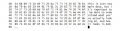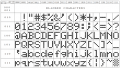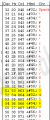# Ascii characters in C language

#### Saumyojit

Joined Feb 20, 2020
16
FROM THE ASCII TABLE:

Decimal Character

48 0
49 1
50 2
51 3
52 4
53 5
54 6
55 7
56 8
57 9

In this table this 0-9 digits are ascii characters for which each of them has corresponding value.

I know that "Everything in memory is treated as numerical values. Computer does not understand ASCII or character values"

1: WE KNOW THAT ASCII code is the numerical representation of charcters then HOW numeric no 0-9 can be treated as characters?They are meant to be integers or numerals right??

2:
char a ='1'; treats 1 as a character
int a = 1;. treats 1 as a integer data type then 1 is a integer or a character?

#### Analog Ground

Joined Apr 24, 2019
460
char a = '1'; /* assigns the integer value 49 to a and a is an 8 bit, integer variable */
int a = 1; /* assigns the integer value 1 to a and a is an integer variable */

'1' is a shorthand way to get the ASCII value for the "1" character. It saves having to look up the value in an ASCII table. It also makes the program easier to read.

#### bug13

Joined Feb 13, 2012
2,002
Addition to what @Analog Ground said, this may help.

These two line are the same:
C:
char a = '1';
char a = 49;

#### MrChips

Joined Oct 2, 2009
29,825
This is one of the most confusing and important concepts in computer science. Master this and you would have made a quantum leap in your computer science understanding. It does not matter what programming language you are using.

•nsaspook

#### Saumyojit

Joined Feb 20, 2020
16
there are ten digits in ascii table ( 0-9) .when i am writing int i=324;does that mean compiler is taking three digits (3,2,4) from the ascii table and considering each of the digits corresponding ascii value and converting them into binary.

#### MrChips

Joined Oct 2, 2009
29,825
But this is the compiler, like a worker in the back room. We don't really know or care what the compiler does.
All we care is that the compile generates computer code that puts integer 324 into a variable i (a memory location or register).

#### djsfantasi

Joined Apr 11, 2010
9,129
there are ten digits in ascii table ( 0-9) .when i am writing int i=324;does that mean compiler is taking three digits (3,2,4) from the ascii table and considering each of the digits corresponding ascii value and converting them into binary.
Update; @MrChips has provided an excellent answer.

Since the output variable is an integer, the variable will contain the decimal variable 324. No character values are used.

When a multi-digit character variable is desired, a character array is used. You would need an array of four 8 bit char values. The array would contain 51, 50, 52 and 0.

The main takeaway is that each type of variable stores values in different ways. And that determines how memory is used. You need to learn each variable type and how it stores values.

#### KeepItSimpleStupid

Joined Mar 4, 2014
5,088
Think about it this way. The compiler can be written in "c".

We can take this concept and make it more abstract and say what if we are a microcontroller and trying to light 7 segment displays in the 88:88 format for time. One way is to encode as BCD (Binary Coded Decimal) digits and have an IC to the conversion to 7-segment. Each digit is strobed.

At the expense of extra bits, we could do the conversion in hardware.

Assembly language doesn't have the ASCII luxury.

Early electronics sent electrical signals that provided BCD numbers throuugh a 1/0 interface, so the BCD digits of say
0001 0011 1001 0000 had to be converted to 1350 decimal.

#### WBahn

Joined Mar 31, 2012
29,494
In this table this 0-9 digits are ascii characters for which each of them has corresponding value.

I know that "Everything in memory is treated as numerical values. Computer does not understand ASCII or character values"

1: WE KNOW THAT ASCII code is the numerical representation of charcters then HOW numeric no 0-9 can be treated as characters?They are meant to be integers or numerals right??

2:
char a ='1'; treats 1 as a character
int a = 1;. treats 1 as a integer data type then 1 is a integer or a character?
Humans often have a hard time making the distinction between what is being represented and how it is being represented.

When I write 42 on a piece of paper, all I have done is make two marks on the paper, one of which looks like a "4" and another, located to its right by a small amount, that looks like a "2". That's the representation. But what I am representing (most likely) is the concept of the value "forty-two". These are very, very distinct and separate things.

so consider the following two lines of code in a text file:

int a = 1;
int a = '1';

If you were to examine the contents of the file and interpret them as a series of 8-bit unsigned decimal values you would find the sequence (depending on whether your system uses LF or CR-LF end of line sequences):

105 110 116 32 97 32 61 49 59 13 10 105 110 116 32 97 32 61 39 49 39 59 13 10

That's all that is in that file -- a sequence of bits that can be interpreted different ways.

What a compiler does is interpret those bits according to a set of rules.

If the rules say to interpret a sequence of bits as an integer, then the compiler will process those bits that way. If the rules say to interpret them as a character, then that's how they will be processed.

#### MrSoftware

Joined Oct 29, 2013
2,144
What's missing is a description of how things are displayed on the screen. To over simplify, when you tell your C program to print number 36, but treat it as an ASCII character, under the hood the program actually looks up the symbol in the ASCII table indexed at 36 (dollar sign, $) and displays that symbol. Here is a link to the table. When you tell your program to print 36 as an integer, it looks up the symbols for the integer values of 3 and 6 and displays those symbols. Remember, in memory there are no little digits 0 1 2 3 4 ... It's actual flip flops or whatever the memory is made from. So when the computer needs to display a value from memory in the way that a human can read it, there is a subsystem that looks that value up in a table of symbols then displays the proper symbols on the screen. So when you tell a function like printf() to display a value, and you tell it to treat the value as an ASCII char, or as an int, the only thing that changes is the symbol it looks up to display. Similarly when you tell the compiler "int a = '1'", the little '' marks tells the compiler to hey go look up the symbol "1" in the ASCII table, and store that index into "a". Where "int a = 1" tells the compiler to just store the explicit value of 1 to "a". #### BobaMosfet Joined Jul 1, 2009 2,102 FROM THE ASCII TABLE: Decimal Character 48 0 49 1 50 2 51 3 52 4 53 5 54 6 55 7 56 8 57 9 In this table this 0-9 digits are ascii characters for which each of them has corresponding value. I know that "Everything in memory is treated as numerical values. Computer does not understand ASCII or character values" 1: WE KNOW THAT ASCII code is the numerical representation of charcters then HOW numeric no 0-9 can be treated as characters?They are meant to be integers or numerals right?? 2: char a ='1'; treats 1 as a character int a = 1;. treats 1 as a integer data type then 1 is a integer or a character? You need to understand the difference between memory, and how you tell your compiler (syntax) to look at information. When you specify a variable 'type', whether it be for char, init, long, unsigned char, short, or whatever- memory doesn't know or care. it only knows _binary_. Your compiler on the other hand needs to know how you want to look at data. And how you specify that data to the compiler, tells it how to place it into memory. If you make a char = '0', your telling the compiler that you want a 48decimal value place into a byte of memory. If you tell the compiler int = 0, you're telling the compiler to place a 0 (zero) decimal value into memory. programming is about talking to a compiler or interpeter using a ruleset called 'grammar'. All you're doing is telling the compiler how _YOU_ want it to view things to satisfy your logic. How data looks in memory:Thread Starter #### Saumyojit Joined Feb 20, 2020 16 Addition to what @Analog Ground said, this may help. These two line are the same: C: char a = '1'; char a = 49; THESE LINES are same in what sense? they will get stored in the memory as 49? #### MrChips Joined Oct 2, 2009 29,825 THESE LINES are same in what sense? they will get stored in the memory as 49? Yes, the end result is the same. If you wish, we can break it down in finer detail what is going on. In essence, the compiler has to read your text file, interpret or decode what you are trying to do according to the syntax rules of the language and generate computer code. How the compiler does this varies from one compiler to another. In most cases the compiler: 1) Reads a text file 2) Preprocesses the text file according to the syntax rules 3) Processes the results of step 2 4) Generates computer instructions executable on the target CPU. It is in step 2 that the compiler recognizes that '1' is the same as 49. Hence in step 4, the compiler generates the same computer code: Put the binary pattern 0011 0001 into a memory location. •bug13 Thread Starter #### Saumyojit Joined Feb 20, 2020 16 Yes, the end result is the same. If you wish, we can break it down in finer detail what is going on. In essence, the compiler has to read your text file, interpret or decode what you are trying to do according to the syntax rules of the language and generate computer code. How the compiler does this varies from one compiler to another. In most cases the compiler: 1) Reads a text file 2) Preprocesses the text file according to the syntax rules 3) Processes the results of step 2 4) Generates computer instructions executable on the target CPU. It is in step 2 that the compiler recognizes that '1' is the same as 49. Hence in step 4, the compiler generates the same computer code: Put the binary pattern 0011 0001 into a memory location. #include<stdio.h> int main() { int x=6; "WHen i am saying 6 as a integer does it takes ascii value of 6(54)or directly converts 6 into binary ? printf("%d",x); return 0; } What about this? Last edited: #### MrChips Joined Oct 2, 2009 29,825 The computer does not know what is 6. Stored in the computer's memory is a pattern of zeros and ones. How many zeroes and ones depends on your definition of int. It can be any number of zeroes and ones (bits). Let us define int as being 16 bits. In the computer is a pattern of zeros and ones: 0000 0000 0000 0110 Now you ask to print this out on the computer screen. You need to tell the computer how to print this using some predefined rules. You (or someone before you) made up the rules. The only thing the computer can do is show you some pictures. So you tell the computer that when it finds 0000 0000 0000 0110 in its memory, show &$#@.
And that is what the computer shows you, a sequence of icons &\$#@.

Here is an example of some pictures (icons) you can put on the computer screen.
Every icon is given a code number. Hence if you want to see 6 on the screen, you have to call up the icon with the code number 011 0110.Where are those rules (instructions)?
The instructions are in the print statement:

printf("%d",x);

We humans made up the rules.
We can make up a rule that says, when ever the computer finds 1111, show the icon F.
The computer has to look up icon code number 100 0110 to find the desired icon to place on the computer screen.

#### Analog Ground

Joined Apr 24, 2019
460
Is now the time to bring up EBCDIC?

#### Kjeldgaard

Joined Apr 7, 2016
476
•nsaspook and MrChips

#### Saumyojit

Joined Feb 20, 2020
16
When you tell your program to print 36 as an integer, it looks up the symbols for the integer values of 3 and 6 and displays those symbols.
Similarly when you tell the compiler "int a = '1'", the little '' marks tells the compiler to hey go look up the symbol "1" in the ASCII table, and store that index into "a". Where "int a = 1" tells the compiler to just store the explicit value of 1 to "a".
@MrSoftware "When you tell your program to print 36 as an integer, it looks up the symbols for the integer values of 3 and 6 and displays those symbols" -> int x=36; What are the corresponding symbols of 3 and 6 . I went through the
ascii table to me the symbols might be end of text(0000011 ) and acknowledge ( 0000110 ) respectively. ??

If you make a char = '0', your telling the compiler that you want a 48decimal value place into a byte of memory. If you tell the compiler int = 0, you're telling the compiler to place a 0 (zero) decimal value into memory

@BobaMosfet
int x=6 ;
char c='6';
printf("\n%c",x);
printf("\n%d",x);

printf("\n%c",c);
printf("\n%d",c);

what am i telling to the compiler for each of variable declaration and 4 output statements?

#### MrSoftware

Joined Oct 29, 2013
2,144
One thing to understand is when "int a=36" and you print the numerical value of "a", the display subsystem takes care of looking up the symbols, it's like a black box to you.

Now if you want to print the characters 3 and 6, here from the ASCII table is what you want. Character "3" is has the ASCII value 51, so if you write the code "char a = '3'" then look at the memory for "a", the memory will hold the actual number 51. You can also write that 51 as 0x33, or 110011 in binary, it's all the same.Run this code, look at the memory and understand what it does:

Code:
#include <stdio.h>

int main()
{
char a = '3';

printf("Variable a holds the ASCII value for the character: %c\n", a);
printf("Variable a holds the numerical value: %i\n", a);

printf("\n\nNow lets print some of the common ASCII symbols (not the entire table)\n");
for(int i = 48; i<=90; i++)
{
printf("Decimal value %i represents ASCII character %c\n", i, i);
}

return 0;
}

#### MrChips

Joined Oct 2, 2009
29,825
@MrSoftware "When you tell your program to print 36 as an integer, it looks up the symbols for the integer values of 3 and 6 and displays those symbols" -> int x=36; What are the corresponding symbols of 3 and 6 . I went through the ascii table to me the symbols might be end of text(0000011 ) and acknowledge ( 0000110 ) respectively. ??

@BobaMosfet
int x=6 ;
char c='6';
printf("\n%c",x);
printf("\n%d",x);

printf("\n%c",c);
printf("\n%d",c);

what am i telling to the compiler for each of variable declaration and 4 output statements?
Go back and read post #15.
Do you understand all of it?